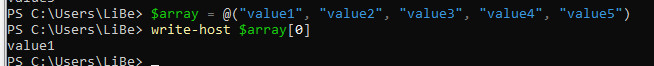## PowerShell Loops and Array

An array stores multiple values, similar to a 2-column table.

## Example

As an example, 5 values could be entered in an array, these can be recalled using ID.

Here is a simple example of an array and its stored values:

 ID Value 0 value1 1 value2 2 value3 3 value4 4 value5

When calling ID 1, the value: "value2" would be output when using the table

## Array

Creating an array works with the following command:

``\$array = @("value1", "value2", "value3", "value4", "value5")``

The values can then be called with the ID 0- ... can be called: `\$array, \$array, . `..

The output to Powershell is done using "Write-Host":

``````\$array = @("value1", "value2", "value3", "value4", "value5")
Write-Host \$array``````
Result:
``````value1
``````The length of the array, i.e. the number of stored values, can be read with `\$array.length`

``````\$array = @("value1", "valuet2", "value3", "value4", "value5")
\$array.length``````
Result:
``````5
``````

The stored values of an array can be processed, for example, using loop.

The following loops (except foreach) have this in common:

• A variable as a counter`(\$i`). This variable is used to count when to exit the loop.
• The counter is assigned a start value `(\$i=0)`.
• The start value is incremented by 1 with each run of the loop`(\$i++)`
• until the final value is reached. The end value is the length of the array`(\$array.length`)
• For checking the final value there is a condition: as long as `\$i` is less than the number of values`(\$i -lt \$array.length`) see also: PowerShell Syntax: compare and nest

## For loop (for loop)

So if we take the above example, we can use For loop to display all values in the array:

``````\$array = @("value1", "value2", "value3", "value4", "value5")
for (\$i=0; \$i -lt \$array.length; \$i++){
Write-Host \$array[\$i]
}``````
Result:
``````value1
value2
value3
value4
value5
``````

The command in detail:

`for (\$i=0; \$i -lt \$array.length; \$i++)`

`\$i=0`... Start value: The variable \$i starts with the value 0

`\$i -lt \$array.length`... Condition: the For loop is executed as long as this condition is met: as long as the variable `\$i` is smaller than `\$array.length`, so as long as `\$i` is smaller than 5.

`\$i++`... Action on one pass of the loop: `\$i++` means increase the value of the variable `\$i` by 1, on each pass of the loop `\$i` will be increased by 1: 0 ... 1 ... 2 .. 3 ....

## while loop (while loop)

We stick to the same example with a while loop.

``````\$array = @("value1", "value2", "value3", "value4", "value5")
\$i=0
while (\$i -lt \$array.length){
Write-Host \$array[\$i]
\$i++
}``````
Result:
``````value1
value2
value3
value4
value5
``````

In the example, the initial value `\$i` is defined before the loop `(\$i=0)`.

The command in detail:

`{ while (\$i -lt \$array.length){`

Inside while (.. stands the condition for the loop to run, as long as it is met, the loop will not exit:

`\$i -lt \$array.length`... as long as `\$i` is less than `\$array.length`

In this example, the variable `\$i` is incremented by 1 within the loop: `\$i++`

### Endless loop -Endless loop

while can be used for an endless loop as follows:

``while(\$true) { #Script block }``

with `break` the endless loop can be left again. The following example runs through the loop until `break` is executed, this happens when `\$i` is no longer less than 10:

``````\$i=0
while(\$true) {
\$i++
write-host \$i
if (\$i -ge 10) {
break
}
}``````
Result:
``````1
2
3
4
5
6
7
8
9
10
``````

## do while loop (do loop)

``````\$array = @("value1", "value2", "value3", "value4", "value5")
\$i=0
do{
write-host \$array[\$i]
\$i++
} while (\$i -lt \$array.length)``````
Result:
``````value1
value2
value3
value4
value5
``````

## foreach

Foreach is used to read all existing values. Foreach does not need a start and end value for execution. The read values are stored in a variable (in the example: `\$i`).

``````\$array = @("value1", "value2", "value3", "value4", "value5")
foreach (\$i in \$array){
Write-Host \$i
}``````
Result:
``````value1
value2
value3
value4
value5
``````

The command in detail:

`foreach (\$i in \$array) . `.. Calling all values of the array`(\$array)`. The variable `\$i` contains the currently read value on each pass.

## foreach Pipe: | %

When used directly in the console, commands are usually simply passed via a pipe "|": A pipe can also be used to pass the array directly to the foreach loop. At this point, the alias % for foreach is often used:

``````@("value1", "value2", "value3", "value4", "value5") | % {
write-host \$_
}``````
Result:
``````value1
value2
value3
value4
value5
``````

The variable "\$_" can be accessed in the loop.

### THANK YOU for your review!

Publication: 2022-05-02 from Bernhard | Übersetzung Deutsch |🔔 | Comments:0